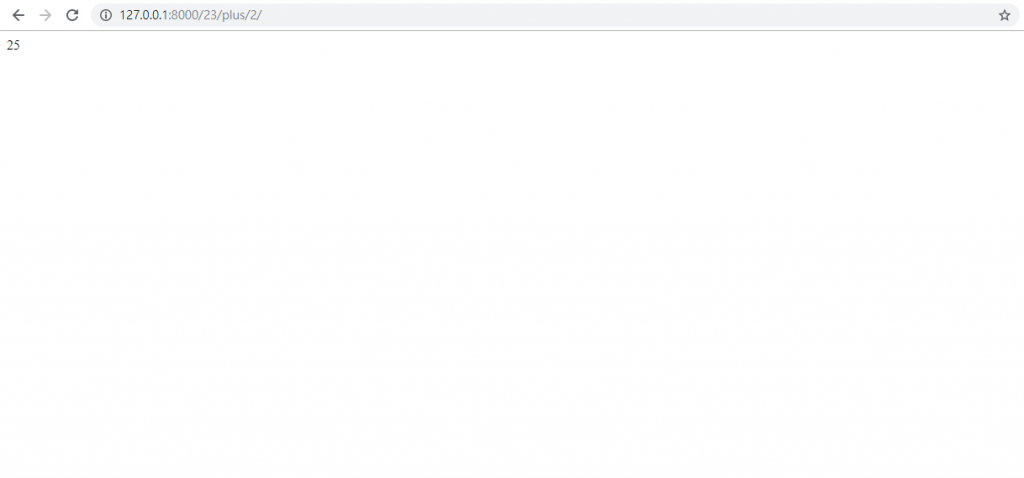#0

## Django學習紀錄 5.視圖與URL[附正規表達式的整理]# 5. 視圖與URL

## 5.1 第一個視圖:媽，我在這裡``````from django.contrib import admin
from django.urls import path
from mysite.views import here # <-加入這行

urlpatterns = [
path('here/', here), # <-加入這行
]
``````

``````from django.http import HttpResponse

def here(request):
return HttpResponse('Mom, I am here!')
``````

HttpResponse是一個基礎的回應物件，可以回傳一個完整的html或字串

``````python manage.py runserver
``````

``````path('here/', here)
``````

``````path('here', here)
``````

``````from django.http import HttpResponse

def here(request):
return HttpResponse('媽，我在這!')
``````

``````# -*- coding: utf-8 -*-
``````

## 5.2 第二個視圖:網址計算機``````from django.contrib import admin
from django.urls import path, re_path # <-引進re_path
from mysite.views import here, add # <-引進add

urlpatterns = [
path('here/', here),
re_path(r'(\d{1,2})/plus/(\d{1,2})', add) # <- 加入這行
]
``````

``````from django.http import HttpResponse

def here(request):
return HttpResponse('媽，我在這!')

def add(request, a, b): # <- 加入這個函式
s = int(a) + int(b)
return HttpResponse(s)
````````````from django.contrib import admin
from django.urls import path, re_path
from mysite.views import here, add, math # <-加入math

urlpatterns = [
path('here/', here),
re_path(r'(\d{1,2})/math/(\d{1,2})/', math), # <-加入這行
]
``````

views.py

``````from django.http import HttpResponse

def here(request):
return HttpResponse('媽，我在這!')

def add(request, a, b):
s = int(a) + int(b)
return HttpResponse(s)

def math(request, a, b): # 加入
a = int(a)
b = int(b)
s = a + b
d = a - b
p = a * b
q = a / b
html = '<html>sum={s}<br>dif={d}<br>pro={p}<br>quo={q}</html>'.format(s=s, d=d, p=p, q=q)
return HttpResponse(html)
``````

/是正常除法 //才是取商
html中 `<br>`是換行

``````re_path(r'(\d{1,2})/math/(\d{1,2})/', math)
``````

``````path('<str:a>/math/<str:b>', math)
``````

str也可以換成int之類的，這樣視圖函式中就不用把參數用`int()`轉換型別了

### 有關正規表達式

regular expression(簡稱regex)

https://regex101.com/

`.` 一個除了換行字元`\n`以外的所有字元
`^` 輸入列的開始
`&` 輸入列的結束
`?` 前一個項目可以出現0次或1次
`[abc]` 代表一個符合a或b或c的任何字元
`[a-z]` 代表一個符合字母a~z的任何字元
`\` 代表後面的字元以一般字元處理
`{m}` 代表前一個項目必須正好出現m次
`{m,}` 代表前一個項目出現次數最少m次，最多無限次
`{m,n}` 代表前一個項目出現次數最少m次，最多n次
`\d` 一個數字字元，相當於```[0-9]`
`^` 對後面的項目做反運算，例如:`[^a-d]`代表除了a、b、c、d外的所有字元
`\D` 一個非數字字元，相當於`[^0-9]`
`\n` 換列字元
`\r` 回列首字元(carriage return)
`\t` tab定位字元
`\s` 空白字元，相當於`[\r\t\n\f]`
`\S` 非空白字元，相當於`[^\r\t\n\f]`
`\w` 一個數字、字母或底線字元，相當於`[0-9a-zA-Z_]`
`\W` 一個非數字、字母或底線字元，相當於`[^\w]`，即`[^0-9a-zA-Z_]`
`*` 前一個項目可以出現0次或無限多次
`+` 前一個項目可以出現1次或無限多次

Email `[a-zA-Z0-9_]+@[a-zA-Z0-9\._]+` guest@kimo.com.tw
URL `https:\/\/[a-zA-Z0-9\.\/_]+` https://www.google.com.tw/

``````re_path(r'([0-9]+\.*[0-9]*)/math/([0-9]+\.*[0-9]*)', math)
``````
``````def math(request, a, b):
a = float(a)
b = float(b)
s = a + b
d = a - b
p = a * b
q = a / b
html = '<html>sum={s}<br>dif={d}<br>pro={p}<br>quo={q}</html>'.format(s=s, d=d, p=p, q=q)
return HttpResponse(html)
``````